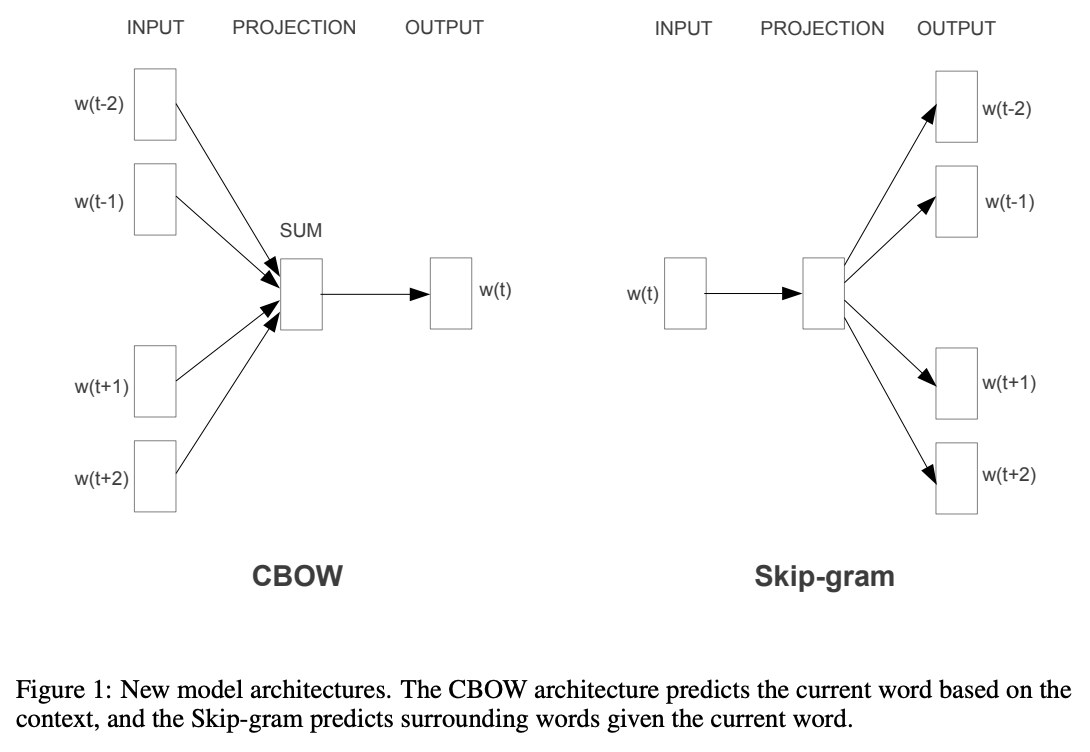# 经典词向量方法回顾

### Course Review

• 课程从最早词向量离散表示（WordNet, OneHot等）介绍到了词向量的稠密表示，主要利用上下文来获取对某个词的表示。
• 具体介绍了Word2Vec及Glove两种词嵌入模型，并介绍了例如hierachical softmax，negative sampling等训练优化方法。
• 介绍了一些常见的词向量评价指标，主要分为intrinsic和extrinsic两大类，前者的典型有词近似类比任务，后者包括一些常见的NLP问题，例如命名实体识别、语言模型等。

### Distributed Representations of Words and Phrases and their Compositionality• 训练时对高频词（例如in,the,a等词意义不大）进行降采样，实验表明这个操作能够同时加快训练效率和提升效果，采样公式为：$P(w_i)=1-\sqrt{\frac{t}{f(w_i)}}$
注：这里是丢弃概率，另外和代码中实现不一致，代码实现更合理，详见第2篇博客的介绍。$f(w_i)$为词频，$t$越小，丢弃概率越大，$t$通常取值在$10^{-5}$附近。

• 考虑到原本的模型对词序没有考虑，可能造成某些词组的表达缺陷，介绍了一种简单的方法将一些单词组合在一起形成phrase，学习phrase的表达，该方法计算公式如下：$score(w_i,w_j)=\frac{count(w_iw_j)-\delta}{count(w_i)\times count(wj)}$
注：$\delta$主要是为了避免将不常见单词组合，score大于预设值的会被组合成一个phrase。通常是在训练数据上以逐渐减小的阈值跑几轮，不断获取长词组。这个思想和当下利用广泛的sentence piece是一样的。

• Skip-Gram模型的原始优化目标：$\frac{1}{T}\sum_{t=1}^{T}\sum_{-c\le j \le c,j\ne 0}\log p(w_{t+j}\mid w_t)$

log里的p概率计算，如果用的是softmax公式，分母归一化项涉及到整个词表，计算复杂度高：$p(w_o\mid w_i)=\frac{{{v}'_{w_o}}^Tv_{w_i}}{\sum_{w=1}^{W}{{v}'_{w_w}}^Tv_{w_i}}$
在这篇paper之前，有人提出用Hierarchical Softmax来替代计算，这是一棵二叉树，每个叶节点就是词表中的每个词，而从根节点到叶节点的二元判别路径就是整个预测路径，将单个概率的计算效率从$O(W)$降低到了$O(log_2(W))$。其计算公式如下：$p(w|w_i)=\prod_{j=1}^{L(w)-1}\sigma([n(w,j+1)=ch(n(w,j))]\cdot {{v}'_{n(w,j)}}^Tv_{w_i}])$
Negative Sampling的方法变换了优化目标，将问题改为判定当前选定的词是不是窗口内的词，一个二元判别问题，损失函数变为：$\log \sigma({{v}'_{w_o}}^Tv_{w_i}) +\sum_{j=1}^{k}\mathbb{E}_{w_j\sim P_n(w)}[\log \sigma(-{{v}'_{w_j}}^Tv_{w_i}))]$
注：有一点值得注意一下，如果采用Hierarchical Softmax后，每个单词只会学习到1个向量表达（输出矩阵仅用于路由，不对应具体词），原本SG模型针对一个单词有2个向量表达。

• 论文实验部分用了大量基于vector的计算近邻任务（特别是单词类别任务，word analogy），实验结果展示了本文最终训练得到的模型的有效性。

### Word2Vec Tutorial – The Skip-Gram Model

– 代码实现中，训练时的高频词降采样公式和paper定义的保留概率$P(w_i)=\sqrt{\frac{t}{f(w_i)}}$ 不同：$P(w_i) = \frac{t}{f(w_i)} + \sqrt{\frac{t}{f(w_i)}}$

• NEG进行负样本选择时的C代码实现很有意思，用了一个包含table_size=100M个元素的数组，里面填充了单词表中各个单词的index，其中某个单词的出现频率用的是下面的计算公式：$P(w_i)=\frac{{f(w_i)}^{3/4}}{\sum_{j=0}^{n}{{f(w_j)}^{3/4}}}$

### GloVe: Global Vectors for Word Representation$J=\sum_{i,j=1}^{V}f(X_{ij})(w^T_iw_j+b_i+b_j-\log X_{ij})^2$$f(x)=\left\{\begin{matrix} (x/x_{max})^\alpha & if\ x\le x_{max}\\ 1 & otherwise \end{matrix}\right.$

### Even More Earlier